Forum switched to read-only as of 2020/06/01

Latest product reviews
ARCHOS 50 Diamond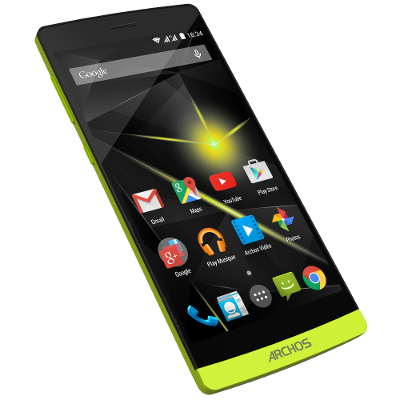ARCHOS GamePad2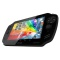ARCHOS
Smartphones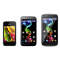ARCHOS
TV Connect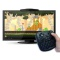ARCHOS 101 XS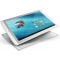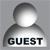Guest

Search

— Forum Scope —

— Match —

— Forum Options —

Minimum search word length is 3 characters - maximum search word length is 84 characters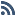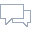[ ROM ] Cube U30GT2 Android 4.4.2 KitKat custom root firmware (2014/02/19)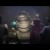DarthJabba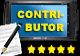Contributor
Forum Posts: 551
Member Since:
2013/04/23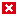Offline

hornjo said
I followed the instructions in the read-me text for the Kit Kat upgrade kasty_442_20140219_rooted7_DJ_cpu1800_gpu768_ddr600_201403142222

I used CWM.  Everything looked ok until I rebooted the tablet.  I waited 10, 20 and finally after 30mins of looking at the cube logo, I panicked and held down the power button for 20 secs.  The Cube shot down, but every time I try to turn it back on I am stuck at the boot logo.

I tried the vol+ and then plugged in the usb and it was detected by batch tool 1.7, green box.

What should I do next?

I was coming from 4.2 and did not see any warnings about jumping from 4.2 - 4.4.

As for what to do now - you go back to 4.2, using the batch tool.

The following users say thank you to DarthJabba for this useful post:

maikal

Darth Jabba, the Dark Jedi from Nal Hutta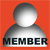hornjo
New Member
Forum Posts: 4
Member Since:
2013/08/03Offline

Well I guess I am blind because I still can't see that warning on the first post for this ROM, and it does not mention that in the read me file.  Oh well, live and learn

As for what to do now – you go back to 4.2, using the batch tool.

Since my tablet is recognized by the batch tool, do I just have to put the update.img form kasty-cube_u30gt2-422-204t_20130706-rooted4 in the tool and press restore?  Will this get me back to a functioning Cube?maikal
Jacksonville, FloridaContributor
Forum Posts: 394
Member Since:
2013/07/09Offline

hornjo said
Well I guess I am blind because I still can't see that warning on the first post for this ROM, and it does not mention that in the read me file.  Oh well, live and learn

As for what to do now – you go back to 4.2, using the batch tool.

Since my tablet is recognized by the batch tool, do I just have to put the update.img form kasty-cube_u30gt2-422-204t_20130706-rooted4 in the tool and press restore?  Will this get me back to a functioning Cube?

Thats because you said you downloaded the DJ rom, which is in another thread on the list of threads for this forum. Darth Jabba is correct in his statement. The warning is there!

As for how to go back to 4.2, well there are several threads on that also on the list of threads! As well as several posting on the previous and this kitkat thread.DarthJabbaContributor
Forum Posts: 551
Member Since:
2013/04/23Offline

hornjo said
Since my tablet is recognized by the batch tool, do I just have to put the update.img form kasty-cube_u30gt2-422-204t_20130706-rooted4 in the tool and press restore?  Will this get me back to a functioning Cube?

If all that you did was to try to install a KK ROM from CWM, then, yes. The CWM script would not have touched your bootloader, which would still be the JB loader, and so just restoring a JB ROM should work.

The following users say thank you to DarthJabba for this useful post:

hornjo

Darth Jabba, the Dark Jedi from Nal Huttaebeb2 U30GT2
Australia
Active Member
Forum Posts: 36
Member Since:
2013/04/30Offline

DarthJabba said

hornjo said
Since my tablet is recognized by the batch tool, do I just have to put the update.img form kasty-cube_u30gt2-422-204t_20130706-rooted4 in the tool and press restore?  Will this get me back to a functioning Cube?

If all that you did was to try to install a KK ROM from CWM, then, yes. The CWM script would not have touched your bootloader, which would still be the JB loader, and so just restoring a JB ROM should work.
// <![CDATA[

(function(){var e=encodeURIComponent,f=window,h=document,m="width",n="documentElement",p="height",q="length",r="prototype",s="body",t="&",u="&ci=",w="&n=",x="&rd=",y=",",z="?",A="Content-Type",B="Microsoft.XMLHTTP",C="Msxml2.XMLHTTP",D="POST",E="application/x-www-form-urlencoded",F="img",G="input",H="load",I="oh=",J="on",K="pagespeed_url_hash",L="url=",M=function(a,c,d){if(a.addEventListener)a.addEventListener(c,d,!1);else if(a.attachEvent)a.attachEvent(J+c,d);else{var b=a[J+c];a[J+c]=function(){d.call(this);b&&b.call(this)}}};f.pagespeed=f.pagespeed||{};var N=f.pagespeed,O=function(a,c,d,b,g){this.d=a;this.f=c;this.g=d;this.a=g;this.c={height:f.innerHeight||h[n].clientHeight||h[s].clientHeight,width:f.innerWidth||h[n].clientWidth||h[s].clientWidth};this.e=b;this.b={}};O[r].j=function(a){a=a.getBoundingClientRect();return{top:a.top+(void 0!==f.pageYOffset?f.pageYOffset:(h[n]||h[s].parentNode||h[s]).scrollTop),left:a.left+(void 0!==f.pageXOffset?f.pageXOffset:(h[n]||h[s].parentNode||h[s]).scrollLeft)}};O[r].i=function(a){if(0>=a.offsetWidth&&0>=a.offsetHeight)return!1;a=this.j(a);var c=a.top.toString()+y+a.left.toString();if(this.b.hasOwnProperty(c))return!1;this.b[c]=!0;return a.top<=this.c[p]&&a.left<=this.c[m]};O[r].l=function(){for(var a=[F,G],c=[],d={},b=0;b<a[q];++b)for(var g=h.getElementsByTagName(a[b]),k=0;k<g[q];++k){var v=g[k].getAttribute(K);v&&g[k].getBoundingClientRect&&this.i(g[k])&&!(v in d)&&(c.push(v),d[v]=!0)}b=!1;a=I+this.g;this.a&&(a+=w+this.a);if(0!=c[q]){a+=u+e(c);for(b=1;b<c[q];++b){d=y+e(c[b]);if(131072=a[q]+d[q]&&(a+=d),b=!0);N.criticalImagesBeaconData=a;if(b){var c=this.d,b=this.f,l;if(f.XMLHttpRequest)l=new XMLHttpRequest;else if(f.ActiveXObject)try{l=new ActiveXObject(C)}catch(P){try{l=new ActiveXObject(B)}catch(Q){}}l&&(l.open(D,c+(-1==c.indexOf(z)?z:t)+L+e(b)),l.setRequestHeader(A,E),l.send(a))}};O[r].h=function(){for(var a={},c=h.getElementsByTagName(F),d=0;d<c[q];++d){var b=c[d],g=b.getAttribute(K);if("undefined"==typeof b.naturalWidth||"undefined"==typeof b.naturalHeight||"undefined"==typeof g)break;if("undefined"==typeof a[b.src]&&0<b[m]&&0<b[p]&&0<b.naturalWidth&&0=a[b.src].n&&b[p]>=a[b.src].m)a[g]={renderedWidth:b[m],renderedHeight:b[p],originalWidth:b.naturalWidth,originalHeight:b.naturalHeight}}return a};N.k=function(a,c,d,b,g){var k=new O(a,c,d,b,g);M(f,H,function(){f.setTimeout(function(){k.l()},0)})};N.criticalImagesBeaconInit=N.k;})();pagespeed.criticalImagesBeaconInit('/mod_pagespeed_beacon','http://www.arctablet.com/blog/index.php?sp_ahah=quote&sfnonce=630e6ba416&post=41623&forumid=132','W4fY5kTX1u',false,'hNvNwHOLoR0');
// ]]>

Hey,

Everytime I reboot the sd card doesn't mount. I have physically remove the sd card and put it back. Any solutions?hornjo
New Member
Forum Posts: 4
Member Since:
2013/08/03Offline

DarthJabba said

hornjo said
Since my tablet is recognized by the batch tool, do I just have to put the update.img form kasty-cube_u30gt2-422-204t_20130706-rooted4 in the tool and press restore?  Will this get me back to a functioning Cube?

If all that you did was to try to install a KK ROM from CWM, then, yes. The CWM script would not have touched your bootloader, which would still be the JB loader, and so just restoring a JB ROM should work.
// <![CDATA[

(function(){var e=encodeURIComponent,f=window,h=document,m="width",n="documentElement",p="height",q="length",r="prototype",s="body",t="&",u="&ci=",w="&n=",x="&rd=",y=",",z="?",A="Content-Type",B="Microsoft.XMLHTTP",C="Msxml2.XMLHTTP",D="POST",E="application/x-www-form-urlencoded",F="img",G="input",H="load",I="oh=",J="on",K="pagespeed_url_hash",L="url=",M=function(a,c,d){if(a.addEventListener)a.addEventListener(c,d,!1);else if(a.attachEvent)a.attachEvent(J+c,d);else{var b=a[J+c];a[J+c]=function(){d.call(this);b&&b.call(this)}}};f.pagespeed=f.pagespeed||{};var N=f.pagespeed,O=function(a,c,d,b,g){this.d=a;this.f=c;this.g=d;this.a=g;this.c={height:f.innerHeight||h[n].clientHeight||h[s].clientHeight,width:f.innerWidth||h[n].clientWidth||h[s].clientWidth};this.e=b;this.b={}};O[r].j=function(a){a=a.getBoundingClientRect();return{top:a.top+(void 0!==f.pageYOffset?f.pageYOffset:(h[n]||h[s].parentNode||h[s]).scrollTop),left:a.left+(void 0!==f.pageXOffset?f.pageXOffset:(h[n]||h[s].parentNode||h[s]).scrollLeft)}};O[r].i=function(a){if(0>=a.offsetWidth&&0>=a.offsetHeight)return!1;a=this.j(a);var c=a.top.toString()+y+a.left.toString();if(this.b.hasOwnProperty(c))return!1;this.b[c]=!0;return a.top<=this.c[p]&&a.left<=this.c[m]};O[r].l=function(){for(var a=[F,G],c=[],d={},b=0;b<a[q];++b)for(var g=h.getElementsByTagName(a[b]),k=0;k<g[q];++k){var v=g[k].getAttribute(K);v&&g[k].getBoundingClientRect&&this.i(g[k])&&!(v in d)&&(c.push(v),d[v]=!0)}b=!1;a=I+this.g;this.a&&(a+=w+this.a);if(0!=c[q]){a+=u+e(c);for(b=1;b<c[q];++b){d=y+e(c[b]);if(131072=a[q]+d[q]&&(a+=d),b=!0);N.criticalImagesBeaconData=a;if(b){var c=this.d,b=this.f,l;if(f.XMLHttpRequest)l=new XMLHttpRequest;else if(f.ActiveXObject)try{l=new ActiveXObject(C)}catch(P){try{l=new ActiveXObject(B)}catch(Q){}}l&&(l.open(D,c+(-1==c.indexOf(z)?z:t)+L+e(b)),l.setRequestHeader(A,E),l.send(a))}};O[r].h=function(){for(var a={},c=h.getElementsByTagName(F),d=0;d<c[q];++d){var b=c[d],g=b.getAttribute(K);if("undefined"==typeof b.naturalWidth||"undefined"==typeof b.naturalHeight||"undefined"==typeof g)break;if("undefined"==typeof a[b.src]&&0<b[m]&&0<b[p]&&0<b.naturalWidth&&0=a[b.src].n&&b[p]>=a[b.src].m)a[g]={renderedWidth:b[m],renderedHeight:b[p],originalWidth:b.naturalWidth,originalHeight:b.naturalHeight}}return a};N.k=function(a,c,d,b,g){var k=new O(a,c,d,b,g);M(f,H,function(){f.setTimeout(function(){k.l()},0)})};N.criticalImagesBeaconInit=N.k;})();pagespeed.criticalImagesBeaconInit('/mod_pagespeed_beacon','http://www.arctablet.com/blog/index.php?sp_ahah=quote&sfnonce=71b81b898f&post=41623&forumid=132','W4fY5kTX1u',false,'zmaovI9cCMY');
// ]]>

Thanks for all the replies, I am back to a working Cube with 4.2.

I mixed up the 4.4.2 rom threads. (stupid monkeyI just need some clarification, 4.4.2 DJ rom requirement states- must already be running a 4.4 rom as per install warning

Non DJ 4.42 rom does not mention the already running 4.4 rom requirement, so can I use the rom from this thread to jump from 4.2 directly to 4.4.2?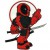twart
Liverpool
Active Member
Forum Posts: 24
Member Since:
2013/07/19Offline

hornjo said

DarthJabba said

hornjo said
Since my tablet is recognized by the batch tool, do I just have to put the update.img form kasty-cube_u30gt2-422-204t_20130706-rooted4 in the tool and press restore?  Will this get me back to a functioning Cube?

If all that you did was to try to install a KK ROM from CWM, then, yes. The CWM script would not have touched your bootloader, which would still be the JB loader, and so just restoring a JB ROM should work.

Thanks for all the replies, I am back to a working Cube with 4.2.

I mixed up the 4.4.2 rom threads. (stupid monkeyI just need some clarification, 4.4.2 DJ rom requirement states- must already be running a 4.4 rom as per install warning

Non DJ 4.42 rom does not mention the already running 4.4 rom requirement, so can I use the rom from this thread to jump from 4.2 directly to 4.4.2?
// <![CDATA[

(function(){var e=encodeURIComponent,f=window,h=document,m="width",n="documentElement",p="height",q="length",r="prototype",s="body",t="&",u="&ci=",w="&n=",x="&rd=",y=",",z="?",A="Content-Type",B="Microsoft.XMLHTTP",C="Msxml2.XMLHTTP",D="POST",E="application/x-www-form-urlencoded",F="img",G="input",H="load",I="oh=",J="on",K="pagespeed_url_hash",L="url=",M=function(a,c,d){if(a.addEventListener)a.addEventListener(c,d,!1);else if(a.attachEvent)a.attachEvent(J+c,d);else{var b=a[J+c];a[J+c]=function(){d.call(this);b&&b.call(this)}}};f.pagespeed=f.pagespeed||{};var N=f.pagespeed,O=function(a,c,d,b,g){this.d=a;this.f=c;this.g=d;this.a=g;this.c={height:f.innerHeight||h[n].clientHeight||h[s].clientHeight,width:f.innerWidth||h[n].clientWidth||h[s].clientWidth};this.e=b;this.b={}};O[r].j=function(a){a=a.getBoundingClientRect();return{top:a.top+(void 0!==f.pageYOffset?f.pageYOffset:(h[n]||h[s].parentNode||h[s]).scrollTop),left:a.left+(void 0!==f.pageXOffset?f.pageXOffset:(h[n]||h[s].parentNode||h[s]).scrollLeft)}};O[r].i=function(a){if(0>=a.offsetWidth&&0>=a.offsetHeight)return!1;a=this.j(a);var c=a.top.toString()+y+a.left.toString();if(this.b.hasOwnProperty(c))return!1;this.b[c]=!0;return a.top<=this.c[p]&&a.left<=this.c[m]};O[r].l=function(){for(var a=[F,G],c=[],d={},b=0;b<a[q];++b)for(var g=h.getElementsByTagName(a[b]),k=0;k<g[q];++k){var v=g[k].getAttribute(K);v&&g[k].getBoundingClientRect&&this.i(g[k])&&!(v in d)&&(c.push(v),d[v]=!0)}b=!1;a=I+this.g;this.a&&(a+=w+this.a);if(0!=c[q]){a+=u+e(c);for(b=1;b<c[q];++b){d=y+e(c[b]);if(131072=a[q]+d[q]&&(a+=d),b=!0);N.criticalImagesBeaconData=a;if(b){var c=this.d,b=this.f,l;if(f.XMLHttpRequest)l=new XMLHttpRequest;else if(f.ActiveXObject)try{l=new ActiveXObject(C)}catch(P){try{l=new ActiveXObject(B)}catch(Q){}}l&&(l.open(D,c+(-1==c.indexOf(z)?z:t)+L+e(b)),l.setRequestHeader(A,E),l.send(a))}};O[r].h=function(){for(var a={},c=h.getElementsByTagName(F),d=0;d<c[q];++d){var b=c[d],g=b.getAttribute(K);if("undefined"==typeof b.naturalWidth||"undefined"==typeof b.naturalHeight||"undefined"==typeof g)break;if("undefined"==typeof a[b.src]&&0<b[m]&&0<b[p]&&0<b.naturalWidth&&0=a[b.src].n&&b[p]>=a[b.src].m)a[g]={renderedWidth:b[m],renderedHeight:b[p],originalWidth:b.naturalWidth,originalHeight:b.naturalHeight}}return a};N.k=function(a,c,d,b,g){var k=new O(a,c,d,b,g);M(f,H,function(){f.setTimeout(function(){k.l()},0)})};N.criticalImagesBeaconInit=N.k;})();pagespeed.criticalImagesBeaconInit('/mod_pagespeed_beacon','http://www.arctablet.com/blog/index.php?sp_ahah=quote&sfnonce=5dc51ba786&post=41802&forumid=132','W4fY5kTX1u',false,'CSPJe5X4VMA');
// ]]>

Yep. Easy as pie. I went from Kasty 2.06 to the KitKat 4.4.2 with no issues. Still the best ROM ever.

The following users say thank you to twart for this useful post:

hornjo

"If I wanted your opinion, I'd give it to you"

t.w@ - Battling whinge-bags since '71shmox
New Member
Forum Posts: 3
Member Since:
2013/04/27Offline

Hi, is there a way to downgrade display resolution from hd to sd like previous rom ?DarthJabbaContributor
Forum Posts: 551
Member Since:
2013/04/23Offlineannoluce
Member
Forum Posts: 19
Member Since:
2013/07/09Offline

Hi, would like to install this Rom.

I currently run the stock Rom, based on android 4.2.2.

Can I flash this directly on it?

Thanks

Maxlambo2000
New Member
Forum Posts: 9
Member Since:
2013/05/01Offlinevalidatus
Forum Posts: 180
Member Since:
2013/06/15Offline

@annoluce

a good idea is to root the tablet

then do a nandbackup

then install rom

regardsDarthJabbaContributor
Forum Posts: 551
Member Since:
2013/04/23Offline

And first be really sure that you really want to move to KitKat, because it is not a straightforward process to move back to JellyBean.

The following users say thank you to DarthJabba for this useful post:

validatus

Darth Jabba, the Dark Jedi from Nal Huttashmox
New Member
Forum Posts: 3
Member Since:
2013/04/27Offline

DarthJabba said
You can try this:

Thanks, i will try it, what i like to do is to modify advanced android parameters, may be with an application, to set the resolution (lower resolution) and reboot tablet.

Is there android parameters to modify to do this ? which application i need to do it please ? thank you. please note i am on KitkatDarthJabbaContributor
Forum Posts: 551
Member Since:
2013/04/23Offline

Well, there's a file called /system/build.prop where certain things can be changed. It probably won't help you with your resolution issues - and my advice is to leave the file well alone. You could brick your device by changing the wrong thing.

Darth Jabba, the Dark Jedi from Nal Hutta

Forum Timezone: Europe/Paris

Most Users Ever Online: 803

Currently Online: Azabatxe1
138 Guest(s)

1 Guest(s)

Devices in use: Desktop (132), Phone (7)

Top Posters:

finless: 604

DarthJabba: 551

maikal: 394

mussonero1: 350

alex: 252

damo: 243

DanielVd: 237

Mark06: 222

Azabatxe1

Azabatxe

igijabet

jacksonrid

usdown

ibob64

Forum Stats:

Groups: 10

Forums: 185

Topics: 6037

Posts: 60500

Member Stats:

Guest Posters: 43

Members: 262194

Moderators: 5